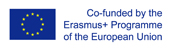This project has been funded with support from the European Commission.
This web site reflects the views only of the author, and the Commission cannot be held responsible for any use which may be made of the information contained therein.

Select language   >   IT EN RO PL LT

### Pythagoras theorem_Video narrating the variety of proofs of the theorem.

Subject taught
- Math

Type of Product
- Videos

Language Skills Developed
- Interaction
- Listening
- Speaking

Transferable/Scientific Skills Developed
The resource helps students to develop their self–directed learning skills while analyzing and comparing different proves of Pythagorean theorem and applying it for solving basic math problems.

Description
General aims:
The teaching resourse introduces the Pythagorean theorem and engages students by narrating of the variety of proofs of the theorem and the possible application of these calculations in Babylon in 1800 B.C., ancient Egypt and India in 800 B.C. long before Pythagoras wrote the theorem.
Linguistic aims:
Students are introduced with key vocabulary: the right angle triange, the square, hypotenuse, legs, side, square korner, tilted, rearrange, rectangles, ratio, equesions. Students use key vocabulary to prove the Pythagorean theorem. Listening to the video and reading the script helps students to improve their pronunciation.
Subject specific aims:
The teaching resource provides several proofs of Pythagorean theorem to demonstrate that a theorem must hold true all the time. One of the proofs provided is by Pythagoras himself and is called proof by rearrangement. Another proof that is presented in the video comes from a Greek mathematician Euclid.
Students are invited to make an experiment with a turntable and three square boxes of equal depth connected to each other around a right triangle. When
the largest square is filled with water and the turntable is spinned, the water from the large square will perfectly fill the two smaller ones. An animated experiment is presented in the video.
After watching this video, students should revise new vocabulary they have learnt. They should be able to define the elements of the right triangle in English, define the Pythagorean theorem and to use key vocabulary to proof the Pythagorean theorem.
This teaching resourse can be used with 12-16 years old students whose level of competence in English (CEFR) is A2, A2+, B1.
Time required to use the resource with the students:
The video is 5 minutes long but it can be stopped several times while watching it, to give students some time to think, to ask questions, participate in a discussion.
After watching and analyzing the video, students, working individually, apply the Pythagorean theorem to solve mathematical problems.
Possible difficulties for the students:
Students who usually find solving mathematical problems difficult may find proving the Pythagorean theorem in English even more difficult.